\$B:#>,\$N0lGU(B

\$BKhF|!":#>,\$N0lGU\$r>e\$2\$F\$\$\$^\$9!#(B
\$B:#>,0{\$`\$b\$N\$K:\$\$C\$?\$i!";29M\$K\$7\$F\$_\$F\$/\$@\$5\$\$!#(B

2023\$BG/(B8\$B7n(B

 1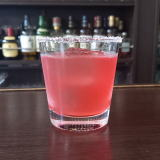(IK0D12W]D^(B 2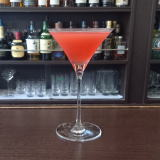(IJ^0F]8^%1/L_(B 3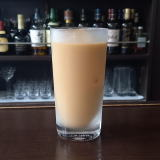(IJ^@LW2(B 4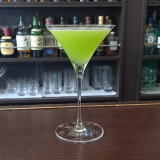(I6XK^1]%8Y0=^(B 5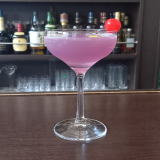(I=M_0=%L']@<^0(B 6 \$B5Y\$_(B 7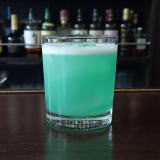(I6^YL%=DX0Q(B 8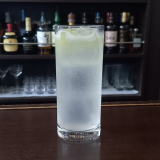(I35/6%X/70(B 9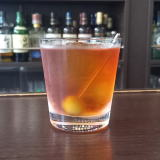(I<^,O26%OC(0F(B 10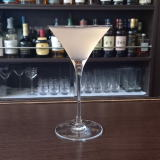(I1[J(B 11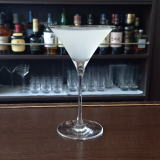(I;]%5]%;^%K^0A(B 12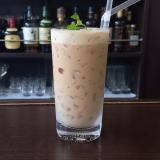(ID0=C/D^%10S]D^(B 13 \$B5Y\$_(B 14(I\2]%:L^W0(B 15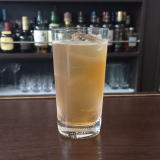(IL^W2D%1]D^%Z2F0(B 16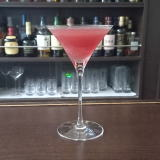(I;]<,2](B 17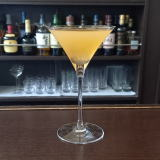(I;O0%D^X0Q(B 18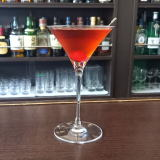(IO]J/@](B 19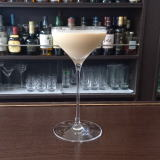(IJ^0J^W(B 20 \$B5Y\$_(B 21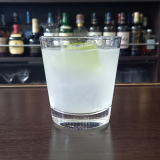(I35/6(B&(IW2Q(B 22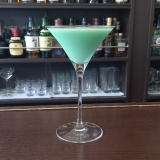(I6XK^1]%O0R2D^(B 23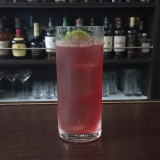(I4Y%C^(1L^[(B 24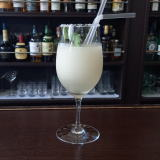(IL[0=^]%OY6^X0@(B 25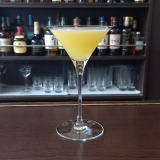(I6XL^(B 26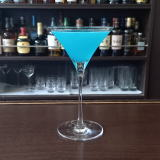(I48?<=D(B 27 \$B5Y\$_(B 28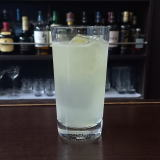(IXS]A*/[%?0@^(B 29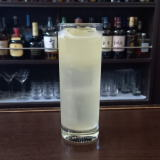(IDQ%:X]=^(B 30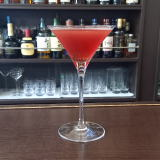(IC^-N^H(B 31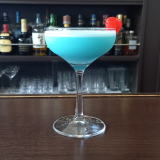(I;O0%J_L-0Q(B